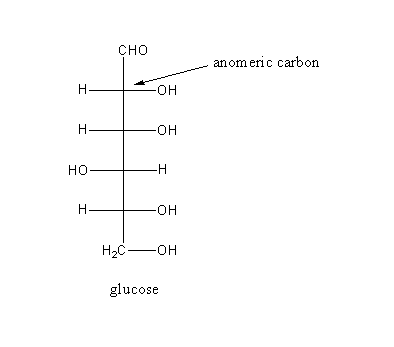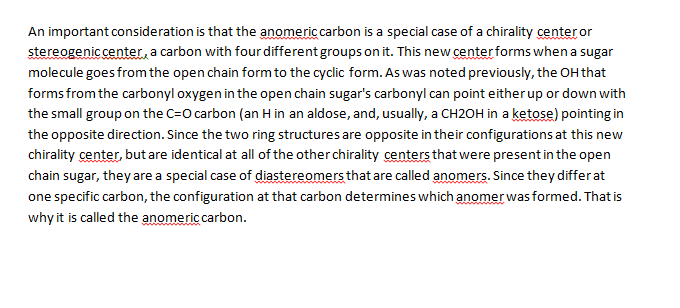In: Chemistry

# In glucose, which carbon is the anomeric carbon?

In glucose, which carbon is the anomeric carbon?

## Solutions

##### Expert Solution## Related Solutions

##### 1. If the C-1 carbon of glucose were labelled with 14C, which of the carbon atoms...
1. If the C-1 carbon of glucose were labelled with 14C, which of the carbon atoms in pyruvate would be labelled after glycolysis? a) the carboxylate carbon b) the carbonyl carbon c) the methyl carbon *** the Answer is c) but I don't understand why, can u plz explain why? 2. How many "high energy" bonds are required to convert oxaloacetate to glucose? ***answer is 4 but I don't understand why, can u plz exapain 3. Which of the following...
##### Which best explains why fatty acids produce more energy per carbon than glucose? a. Glucose must...
Which best explains why fatty acids produce more energy per carbon than glucose? a. Glucose must proceed through more pathways to yield ATP b. Fatty acids are already located in the mitochondrial matrix c. Glucose molecules are already partially oxidized d. All of the above
##### The reaction of glucose, C6H12O6, to carbon dioxide and water is a common reaction in the...
The reaction of glucose, C6H12O6, to carbon dioxide and water is a common reaction in the body. C6H12O6 (s) + _6__ O2 (g) → __6__ CO2 (g) + __6__ H2O (g) If you eat 15.0 g of glucose from some candy you eat, how many grams of carbon dioxide will you produce? If the density of water at body temperature (37 ⁰C) is 0.9933 g/mL, how many mL of water is produced from this reaction?
##### Consider the formation of glucose from carbon dioxide and water (i.e., the reaction of the photosynthetic...
Consider the formation of glucose from carbon dioxide and water (i.e., the reaction of the photosynthetic process): 6CO2(g)+6H2O(l)→C6H12O6(s)+6O2(g) . The following table of information will be useful in working this problem: T= 298 K CO2(g) H2O(l) C6H12O6(s) O2(g) ΔH∘f (kJ mol−1) -393.5 -285.8 -1273.1 0.0 S∘ (J mol−1 K−1) 213.8 70.0 209.2 205.2 C∘P,m (J mol−1 K−1) 37.1 75.3 219.2 29.4 Calculate the entropy change for this chemical system at T = 298 K. Calculate the enthalpy change for this...
##### A sample of glucose, C6H12O6 has a mass of 300.0g. What mass of carbon atoms are...
A sample of glucose, C6H12O6 has a mass of 300.0g. What mass of carbon atoms are in the sample? What mass of hydrogen atoms are in the sample? What mass of oxygen atoms are in the sample?
##### Consider the formation of glucose from carbon dioxide and water (i.e., the reaction of the photosynthetic...
Consider the formation of glucose from carbon dioxide and water (i.e., the reaction of the photosynthetic process): 6CO2(g)+6H2O(l)→C6H12O6(s)+6O2(g). The following table of information will be useful in working this problem: T= 298 K CO2(g) H2O(l) C6H12O6(s) O2(g) ΔH∘f (kJ mol−1) -393.5 -285.8 -1273.1 0.0 S∘ (J mol−1 K−1) 213.8 70.0 209.2 205.2 C∘P,m (J mol−1 K−1) 37.1 75.3 219.2 29.4 A. Calculate the entropy change for this chemical system at T = 298 K. B. Calculate the enthalpy change for...
##### 6. Glucose is completely oxidized to carbon dioxide with the concomitant production of several reduced electron...
6. Glucose is completely oxidized to carbon dioxide with the concomitant production of several reduced electron carrier molecules. Oxidative phosphorylation ensures the regeneration of these electron carrier molecules. A. Cell Y is able to carry out glycolysis, citric acid cycle, and oxidative phosphorylation. However, a certain mutation in ATP synthase allows the passage of H+ ions but does not produce ATP. When grown in aerobic conditions, would you expect Cell Y to generate a little less OR a little more...
##### In photosynthesis, green plants convert carbon dioxide and water into glucose (C6H12O6) according to the following...
In photosynthesis, green plants convert carbon dioxide and water into glucose (C6H12O6) according to the following equation: 6CO2(g)+6H2O(l)→ C6H12O6(aq)+6O2(g). Estimate ΔH for the reaction using bond dissociation energies from Table 7.1. Give your answer in kcal/mol and kJ/mol. C6H12O6 has five C−C bonds, seven C−H bonds, seven C−O bonds, and five O−H bonds. Express your answers as integers separated by a comma.
##### Problem 5.1 Consider the formation of glucose from carbon dioxide and water (i.e., the reaction of...
Problem 5.1 Consider the formation of glucose from carbon dioxide and water (i.e., the reaction of the photosynthetic process): 6CO2(g)+6H2O(l)?C6H12O6(s)+6O2(g). The following table of information will be useful in working this problem: T= 298 K CO2(g) H2O(l) C6H12O6(s) O2(g) ?H?f (kJ mol?1) -393.5 -285.8 -1273.1 0.0 S? (J mol?1 K?1) 213.8 70.0 209.2 205.2 C?P,m (J mol?1 K?1) 37.1 75.3 219.2 29.4 Part A Calculate the entropy change for this chemical system at 313 K . Express your answer to...
##### Which correctly describes the process by which carbon black is produced? (A) Carbon black will form...
Which correctly describes the process by which carbon black is produced? (A) Carbon black will form from any carbon-containing substance held at sufficiently high temperature and pressure. (B) Carbon black forms during the incomplete combustion of wood. (C) Carbon black forms during the incomplete combustion of hydrocarbons like methane. (D)Carbon black forms during the incomplete combustion of coal.